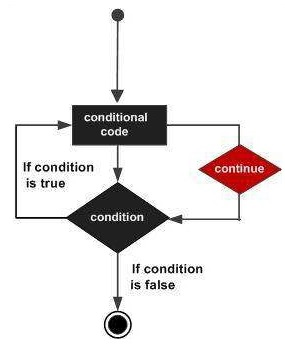# Swift - Continue Statement

The continue statement in Swift 4 tells a loop to stop what it is doing and start again at the beginning of the next iteration through the loop.

For a for loop, the continue statement causes the conditional test and increments the portions of the loop to execute. For while and do...while loops, the continue statement causes the program control to pass to the conditional tests.

## Syntax

The syntax for a continue statement in Swift 4 is as follows −

```continue
```

## Flow Diagram## Example

```var index = 10

repeat {
index = index + 1
if( index == 15 ){
continue
}
print( "Value of index is \(index)")
} while index < 20
```

When the above code is compiled and executed, it produces the following result −

```Value of index is 11
Value of index is 12
Value of index is 13
Value of index is 14
Value of index is 16
Value of index is 17
Value of index is 18
Value of index is 19
Value of index is 20
```
swift_loops.htm